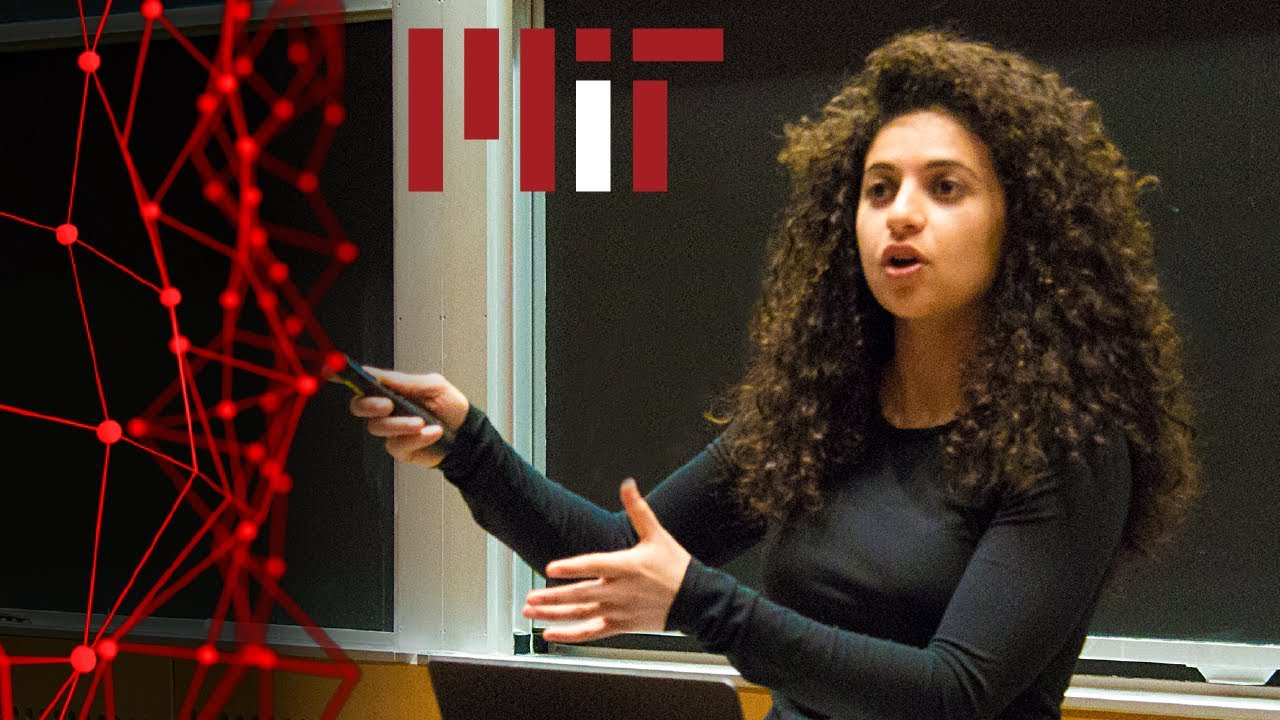# tensorflow tutorial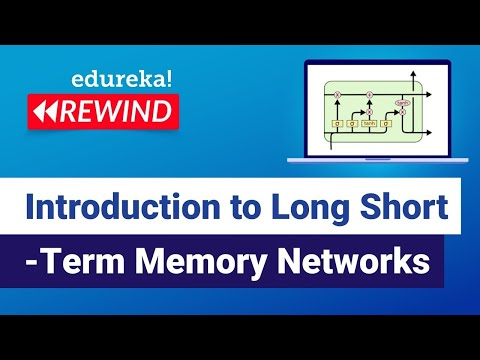Neural Networks

#### Introduction to Long Short-Term Memory Networks (LSTM)

This Edureka LSTM Explained video will help you in understanding why we need Recurrent Neural Networks (RNN) and what exactly it is. It also explains few issues with training a Recurrent Neural Network and how to overcome those challenges using LSTMs.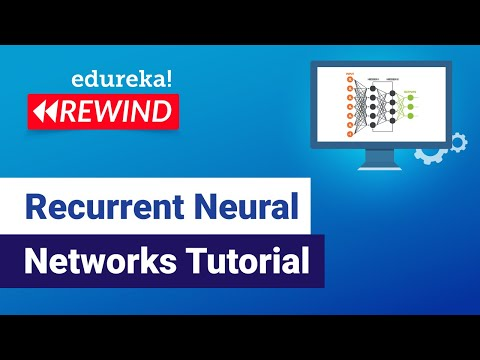Neural Networks

#### Recurrent Neural Networks Tutorial

This Edureka Recurrent Neural Networks tutorial video will help you in understanding why we need Recurrent Neural Networks (RNN) and what exactly it is. It also explains few issues with training a Recurrent Neural Network and how to overcome those challenges using LSTMs. The last section includes a use-case of LSTM to predict the next […]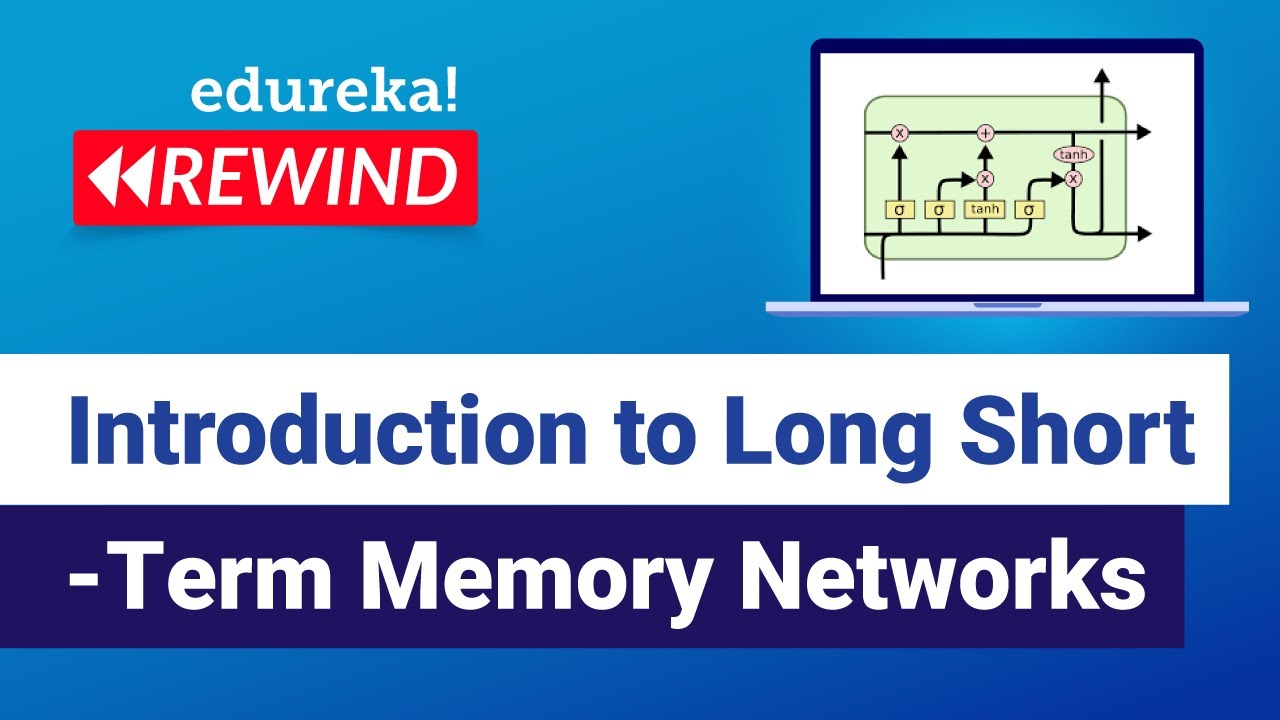Neural Networks

#### Introduction to Long Short-Term Memory Networks (LSTM)

This Edureka LSTM Explained video will help you in understanding why we need Recurrent Neural Networks (RNN) and what exactly it is. It also explains few issues with training a Recurrent Neural Network and how to overcome those challenges using LSTMs.Natural Language Processing TensorFlow

#### Text Classification Using BERT & Tensorflow

Using BERT and Tensorflow 2.0, we will write simple code to classify emails as spam or not spam. BERT will be used to generate sentence encoding for all emails and after that we will use a simple neural network with one drop out layer and one output layer.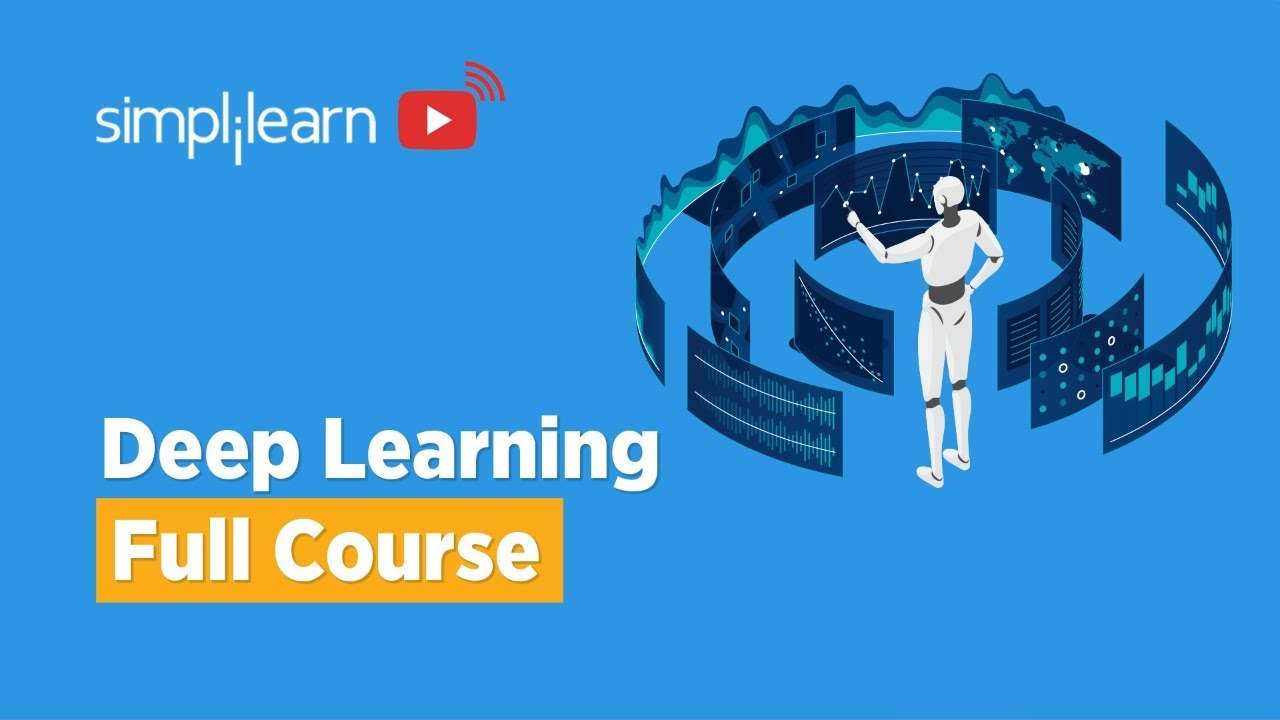Deep Learning

#### Free Deep Learning Full 6 Hour Course

Simplilearn posted this full six hour deep learning course that you can take for free!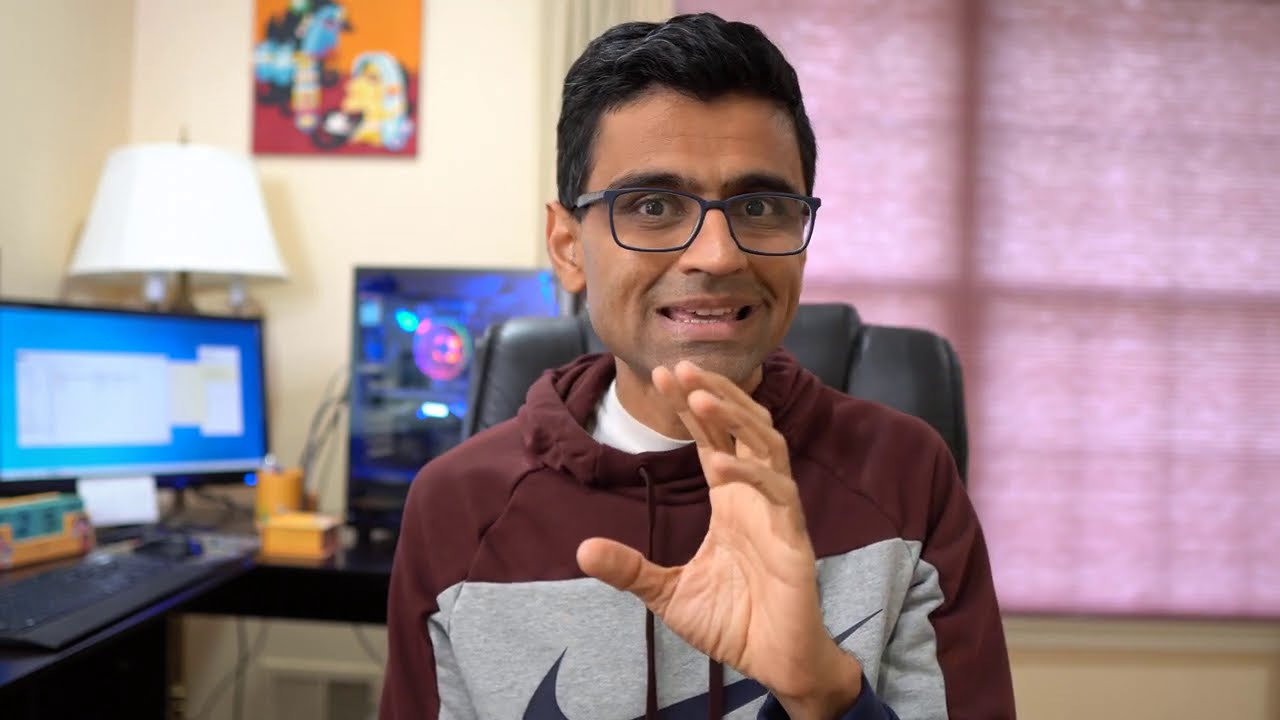Data Science Machine Learning

#### How to Use Data Augmentation to Address Overfitting

codebasics explains how to use data augmentation to address overfitting. When we don’t have enough training samples to cover diverse cases in image classification, often CNN might overfit. To address this we use a technique called data augmentation in deep learning. Data augmentation is used to generate new training samples from current training set using […]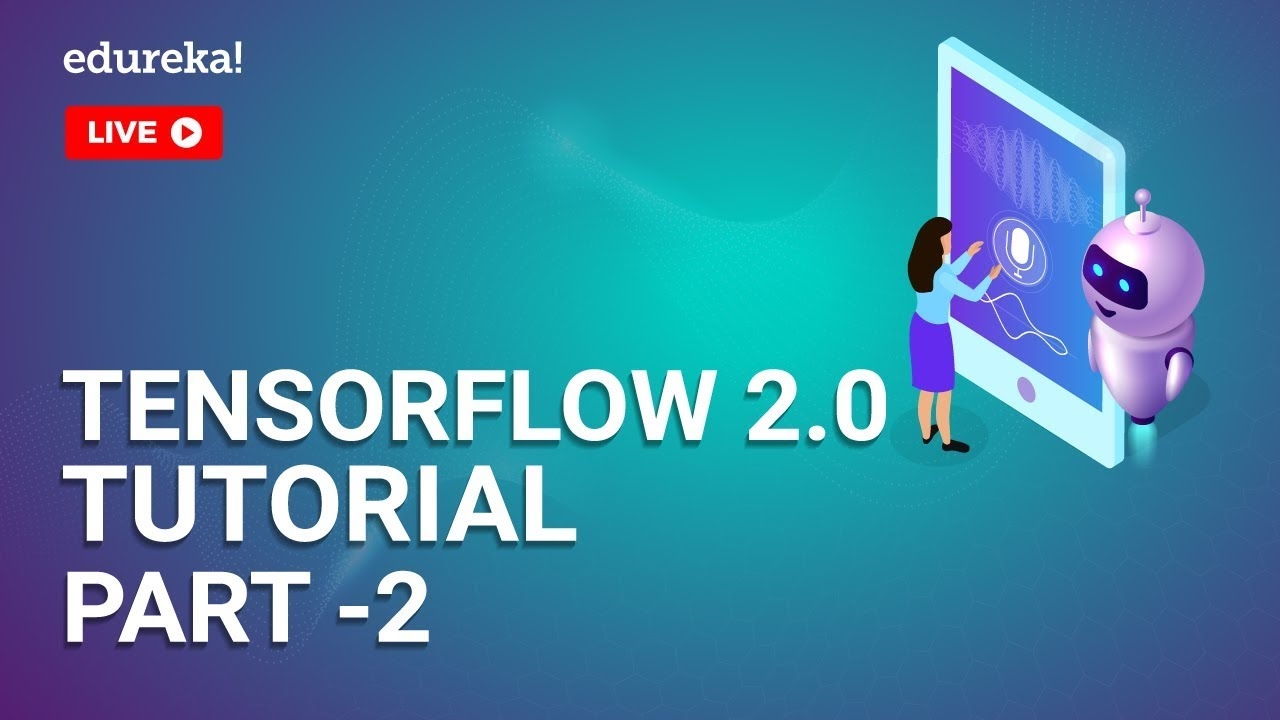TensorFlow

#### TensorFlow 2.0 Tutorial – Part 2: Image Classification

edureka! recently live streamed this tutorial on image classification in TensorFlow 2.0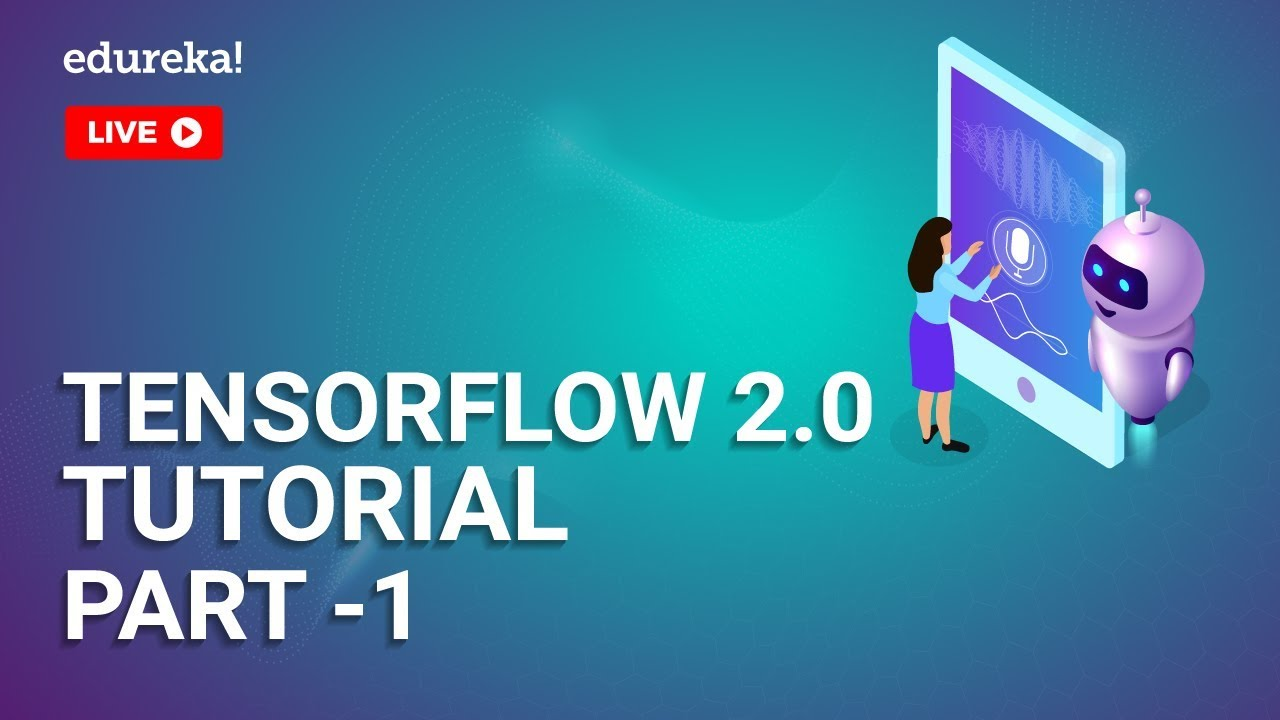TensorFlow

#### TensorFlow 2.0 Tutorial – Part 1

edureka! recently streamed this TensorFlow 2.0 tutorial.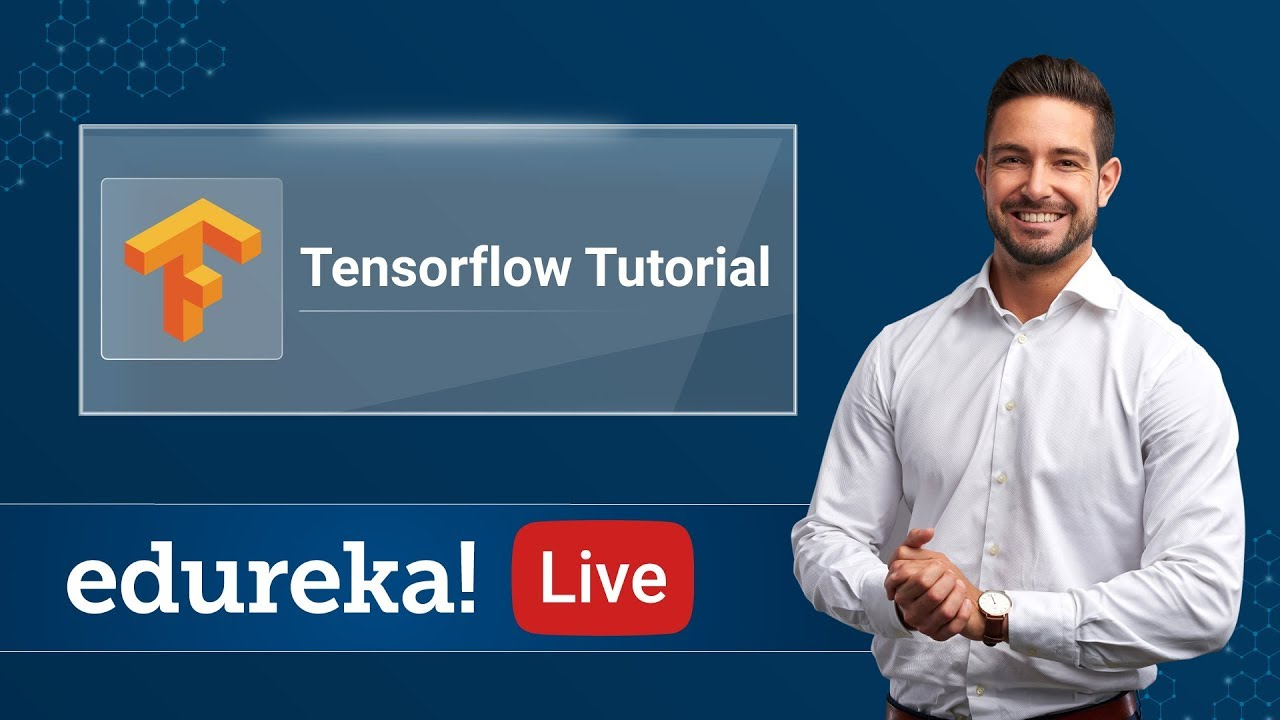Deep Learning TensorFlow

#### Deep Learning Using TensorFlow

edureka! recently live streamed this Deep Learning using TensorFlow tutorial and it’s well worth watching.